# Convergence of measures

(diff) ← Older revision | Latest revision (diff) | Newer revision → (diff)

A concept in measure theory, determined by a certain topology in a space of measures that are defined on a certain-algebra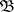of subsets of a spaceor, more generally, in a spaceof charges, i.e. countably-additive real or complex functions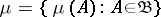, defined on sets from. The following are the most commonly used topologies in the subspace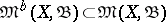consisting of bounded charges, i.e. charges for which,.

1) Inthe norm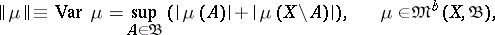called the variation of the charge, is introduced. The convergence of a sequence of charges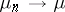,, to a chargein this norm is called convergence in variation.

2) In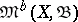the ordinary weak topology is examined: Convergence of a sequence of charges,, in this topology (weak convergence) means that for any continuous linear functionon,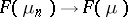,. This convergence is equivalent to the fact that the sequence of charges is bounded,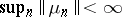, and that for any set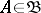the sequence of values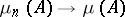,. Weak convergence of a sequence of charges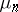,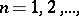implies convergence of the integrals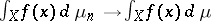,, for any bounded functiononthat is measurable with respect to the-algebra.

3) Whenis a topological space andis its Borel-algebra, a topology is examined inwhich is also called the weak topology (or sometimes the narrow topology). It is defined as the weakest of the topologies inrelative to which all functionals of the form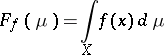are continuous, whereis an arbitrary bounded continuous function on. This topology is weaker than the previous one, and convergence of a sequence of charges,, relative to it (weak or narrow convergence) is equivalent to the convergence of the values,, for any Borel setfor which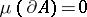, where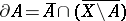and the operation of closure of a set is denoted by the bar.

4) Whenis a locally compact topological space (and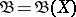is a Borel-algebra) inthe so-called wide topology is examined: the convergence of a sequence of charges,(wide convergence), means convergence of the functionals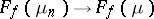,, for any continuous functionwith compact support. This topology is weaker than the weak topology in. An analogous topology is defined naturally in the wider space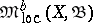of locally bounded charges, i.e. charges such that for any pointthere is a neighbourhoodin which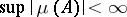,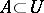,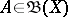.

How to Cite This Entry:
Convergence of measures. Encyclopedia of Mathematics. URL: http://encyclopediaofmath.org/index.php?title=Convergence_of_measures&oldid=14546
This article was adapted from an original article by R.A. Minlos (originator), which appeared in Encyclopedia of Mathematics - ISBN 1402006098. See original article